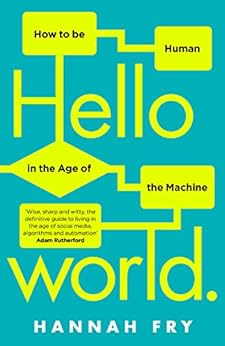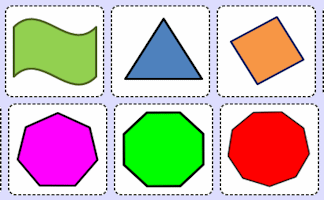Show Mirror Image

Show Mirror Image

Show Mirror Image

Show Mirror Image

Show Mirror Image

Show Mirror Image

Show Mirror Image

Show Mirror Image

The bottom half of some symmetrical calculations are shown above. Can you work out the answers?
Can you make up some other calculations where the calculation and the answer both have horizontal lines of symmetry?

[Teacher: pull down the mirror at the top of the screen to show the top halves of the calculations]

A Mathematics Lesson Starter Of The Day

Share
• Aimee Hamilton, Harrytown Catholic High School
•
• 80+101=181
• Abigail Latham, Swinton
•
• Hello.
Im Abigail Latham From St. Charles Rc Primary School Swinton Manchester
And I Found That 300+100+300+300=1000
30+30+30+10=100
10x10=100
• Abz :D, St Charles Rc Primary
•
• -100+300+300+300=800
800x10=8000
0x10=0
300.08x10=3000.8
=]:D
• Alice K, St Matts
•
• 100 + 88 +111 +1 = 300.
• Primary 7, Bargeddie Primary School
•
• We enjoyed this starter, especially since one of our groups are studying symmetry...
Here are some of our examples:
333 - 333 + 1 = 1
33 x 3 + 1 = 100
11 x 3 = 33
111 - 111 + 8 = 8
Callum in our class also noticed that multiplying any combination of multiples of 10 would give a symmetrical answer:
e.g. 1000 x 1000, 1 000 000 000 x 1000, 10 x 100 000 000 000 etc.
• Mr. T, Gartree High School
•
• Good for younger groups like year 7s since the sums are pretty straight forward but they liked trying to work out how to reflect the digits.
• Tomiloba Awosika, Bishop Challoner School
•
• Tomiloba from Bishop Challoner School 8B3 has come up with an example - 300 + 300 + 300 - 100 = 800.
• Prendergast, Prendergast 9.1 Maths
•
• 11 + 11 + 11 = 33
11 + 11 + 38 + 10 + 10 = 80
8 x 1 = 8
11 x 3 = 33
11 x 8 = 88
13 + 18 = 31
3 x 1 = 3
1 + 1 + 1 + 1 + 1 + 1 + 1 + 1 = 8
1 + 1 + 1 = 3
symmtrical number x 0 = 0.
• Elliot, 7X3WO
•
• 10+0=10.
• Octavia Pullman, London School Of Rock, London
•
• 30+8= 38.

How did you use this starter? Can you suggest how teachers could present or develop this resource? Do you have any comments? It is always useful to receive feedback and helps make this free resource even more useful for Maths teachers anywhere in the world.

If you don't have the time to provide feedback we'd really appreciate it if you could give this page a score! We are constantly improving and adding to these starters so it would be really helpful to know which ones are most useful. Simply click on a button below:

Excellent, I would like to see more like this
Good, achieved the results I required
Satisfactory
Didn't really capture the interest of the students
Not for me! I wouldn't use this type of activity.

This starter has scored a mean of 3.1 out of 5 based on 437 votes.

Previous Day | This starter is for 10 March | Next Day

 l l + 38 = 49 88 x 33 = 2904 838 - 383 = 455 l 8 l x l l = 1991 3 l + 3 l + 3 l = 93 l 0 x l 0 l + l l l = 1121 l 8 l x l 3 l - 80 = 23631 l 380 + 38 - 83 = 1335

 Your next challenge is to make an equation with a horizontal and a vertical line of symmetry or a mathematical ambigram Ambigrams were made famous in Dan Brown's novel "Angels and Demons"

Your access to the majority of the Transum resources continues to be free but you can help support the continued growth of the website by doing your Amazon shopping using the links on this page. Below is an Amazon search box and some items chosen and recommended by Transum Mathematics to get you started.

Hello World

You are buying a (driverless) car. One vehicle is programmed to save as many lives as possible in a collision. Another promises to prioritize the lives of its passengers. Which do you choose?

Welcome to the age of the algorithm, the story of a not-too-distant future where machines rule supreme, making important decisions – in healthcare, transport, finance, security, what we watch, where we go even who we send to prison. So how much should we rely on them? What kind of future do we want?

Hannah Fry takes us on a tour of the good, the bad and the downright ugly of the algorithms that surround us. In Hello World she lifts the lid on their inner workings, demonstrates their power, exposes their limitations, and examines whether they really are an improvement on the humans they are replacing. more...Teacher, do your students have access to computers?Do they have iPads or Laptops in Lessons? Whether your students each have a TabletPC, a Surface or a Mac, this activity lends itself to eLearning (Engaged Learning).Here a concise URL for a version of this page without the comments.

Transum.org/go/?Start=March10

Here is the URL which will take them to an activity about rotational symmetry.

Transum.org/go/?to=rotateFor All: